# Frequency of oscillation

A weight of 1 kg was suspended from the spring and the spring was extended by 1.5 cm. Determine the frequency of the natural oscillation of the resulting oscillator.

f =  4.0701 Hz

### Step-by-step explanation: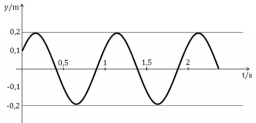Did you find an error or inaccuracy? Feel free to write us. Thank you!Tips to related online calculators
Do you want to convert mass units?
Do you want to convert time units like minutes to seconds?

## Related math problems and questions:

• The oscillation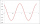The oscillation of a mechanical oscillator can be used to measure weight. A body of unknown weight is suspended on a spring with a stiffness of 20 N times m. The resulting oscillator oscillates with a frequency of 1.6 Hz. Find the weight of the body.
• Cuboid 5Calculate the mass of the cuboid with dimensions of 12 cm; 0.8 dm and 100 mm made from spruce wood (density = 550 kg/m3).
• Dynamometer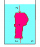What is the volume of a body that stretches a dynamometer in air, on which it is suspended by a force of 2.5 N, and if it is immersed in alcohol with a density of 800 kg/m ^ 3, does it tension the dynamometer with a force of 1.3 N?
• The swing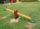To swing the two girls. Aneta weight 45 kg and Simon 35 kg weight. How far should sit Simon from the middle of swing so it is balanced, if we know that Aneta is sitting at distance 1,5m? How far are girls sitting apart?
• The glass1 m3 of glass weighs 2600 kg. Calculate the weight of the glass glazing panel with dimensions of 2.5 m and 3.8 m if the thickness of the glass is 0.8 cm
• CanisterGasoline is stored in a cuboid canister having dimensions 44.5 cm, 30 cm, 16 cm. What is the total weight of a full canister when one cubic meter of gasoline weighs 710 kg and the weight of empty canister is 1.5 kg?
• Harmonic oscillator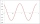Calculate the total energy of a body performing a harmonic oscillating motion if its mass is 200 g, the amplitude of the deflection is 2 cm and the frequency is 5 Hz. Round the result to 3 decimal places.
• Center of gravityThe mass points are distributed in space as follows - specify by coordinates and weight. Find the center of gravity of the mass points system: A1 [1; -20; 3] m1 = 46 kg A2 [-20; 2; 9] m2 = 81 kg A3 [9
• A stone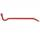There is a stone weighing 60 kg on the billet. The distance from the support point to the stone is 20cm. The length of the billet is 1m. Determine the force exerted by the hand at the end of the billet.
• Brass tubeThe outer perimeter of brass tube (ρ = 8.5 g/cm3) is 38 cm. Its mass is 5 kg, length 54 cm. What is the pipe wall thickness?
• Copper wireWhat is the weight in kg of copper wire 200 m long with a diameter of 0.6 cm, if the density of copper is 8.8 kg?
• Glass doorWhat is the weight of glass door panel 5 mm thick height 2.1 meters and a width of 65 cm and 1 cubic dm of glass weighs 2.5 kg?
• Vegan shopTo the shops brought together 23.2 kg of goods, fruits, vegetables and nuts. fruit was 4.7 kg more than vegetables, nuts was 1.5 kg less than the fruit. Determine the amount of fruits, vegetables and nuts.
• Chained winch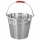When digging a well, a chained winch is used to balance the material. An empty shackle weighs 8 kg, a full 64 kg. What force must the worker exert on the crank handle when pulling the shackle with a uniform movement from a depth of 5 m if the shaft diamet
• Hollow sphereThe steel hollow sphere floats on the water plunged into half its volume. Determine the outer radius of the sphere and wall thickness, if you know that the weight of the sphere is 0.5 kg and the density of steel is 7850 kg/m3
• Foot area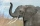Which animal will leave deeper footstep: an elephant weighing 5 tons with a total foot area of 0.5 square meters, or a gazelle weighing 10 kg and a foot area of 50 cm square?
• BricksOpenings in perforated bricks occupy 10% and brick has dimensions 30 cm, 15 cm and 7.5 cm. Calculate a) the weight of a perforated bricks, if you know that the density of the full brick material is p = 1800 kg/m3 (1.8 kg/dm3) b) the number of perforated